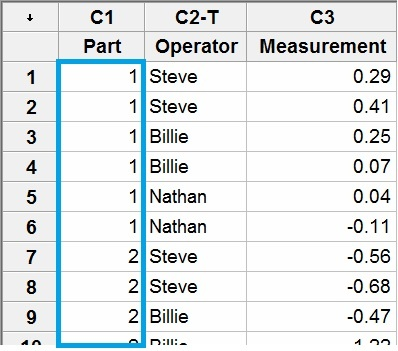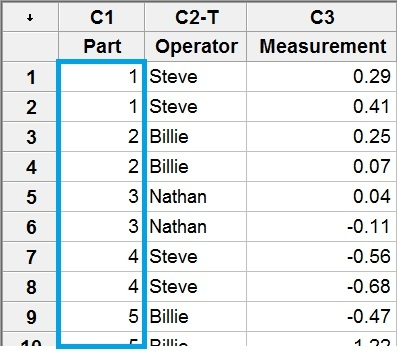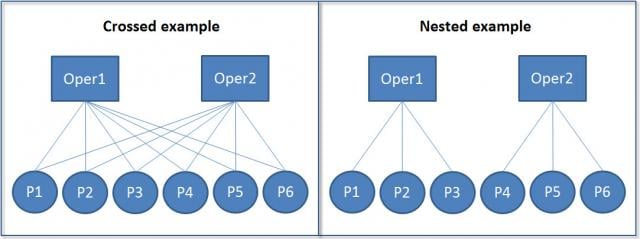Measurement systems analysis (MSA) is essential to the success of any data analysis. If you cannot rely on the tool you’re using to take measurements, then why bother collecting data to begin with? It would be like trying to lose weight while relying on a scale that doesn’t work. What’s the point in weighing yourself?

Minitab Statistical Software offers many types of tools that you can use to assess your measurement system, including:

• Gage R&R Study (Crossed)
• Gage R&R Study (Nested)
• Attribute Agreement Analysis

## Destructive Testing Defined

In MSA studies for continuous measurements (e.g. weight, length, volume) using non-destructive testing, each partcan be measured repeatedly. In this case, we can use crossed Gage studies. However, sometimes we must conduct an MSA where the test required to take the measurement destroys the object or physically changes the characteristic that is being measured. Examples include impact testing and chemical analysis. Picture frozen chickens being hurled at aircraft windshields or testing the amount of force required to open a bag of potato chips.

So what analysis do we use when the test is destructive? Like many questions that arise where statistics are concerned, the answer is, of course, “it depends.”

## Crossed Gage R&R for Destructive Testing

Suppose you are conducting a Gage R&R study with 3 operators where each operator measures each part twice. This requires 3x2=6 measurements per part. Suppose for your destructive test you can obtain at least 6 specimens that are sufficiently similar to be considered the same part. Even though the 6 specimens are truly not the same part, as long as they’re similar enough to be considered the same part, then you can use a crossed Gage study just like you would for a non-destructive test.

This homogeneity assumption is critical to obtain meaningful results because Minitab presumes they are the same, identical part. In Minitab, you label the parts with the same ID (e.g., Part 1) even though they are physically different parts, as shown in the worksheet.Note: The order of the measurements shown in the Minitab worksheet does not reflect the order in which the data should be collected. Parts should be measured in a random order to reduce time-related biases, such as operator fatigue or instrument drift. Randomizing the part order also ensures that operators do not easily recall previous measurements for the parts.

## Nested Gage R&R for Destructive Testing

Suppose again that you are conducting a Gage R&R study for a destructive test with 3 operators and 2 replicates per part. But suppose it is not feasible to obtain 6 specimens that are similar enough to be considered the same part, rather only 2 specimens. Then in this case, you must use a nested analysis.Each operator measures a different set of parts. Therefore, each part is said to be “nested” within operator rather than “crossed” since each part is unique to an operator. Let’s look at a diagram for another view of crossed versus nested studies.Presuming each operator is going to measure each part twice, the crossed example shows 2 operators each measuring the same 6 parts for a total of 24 measurements (2 operators x 6 parts x 2 replicates). In the nested scenario where we again have 2 replicates, each part (e.g. P1) actually represents 2 physically different parts that are similar enough be considered identical. With 2 operators each measuring 3 parts twice, there would be a total of 12 measurements (2x3x2) in this case.

## Crossed vs. Nested Summary

If you want to conduct a reliable statistical analysis, then assessing your measurement system is critical. In the case of destructive testing, your first step is to choose between a crossed and nested study. Ask yourself:

• How many parts can I obtain that are similar enough to be considered identical?
• How many operators will participate in the study?
• How many times is each operator going to measure the “same” part? Two times? Three times? Etc.

Once you have the answers to these questions, you can then determine whether a crossed or nested study is more appropriate. Regardless of which you choose, both analyses will provide meaningful results, and both can be easily conducted using Minitab. For specific Gage R&R examples using Minitab, see our articles on crossed and nested studies.